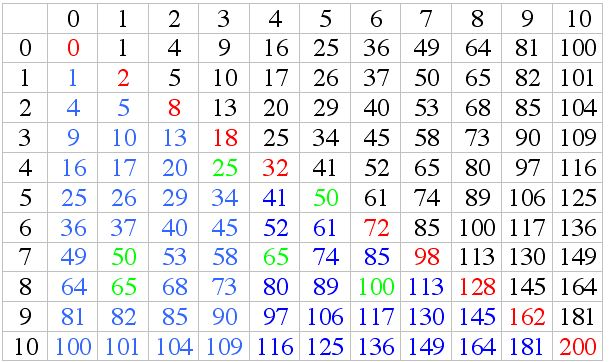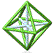Bowen’s morning class notes: July 2, 2002 by Art MabbottNo multiples of 11, yet. If p is prime, and p ≠ a2 + b2, then no multiples of p are in the list until p2. Primes p = 3n + 3 don’t show up in the list. Any number x = 4n + 3 doesn’t show on the list. If a number can be written in more than one way, then it’s a multiple of 5. (up to 53). Closed under multiplication. If n = a2 + b2, and m = c2 + d2, then nm = (   )2 + (   )2 Powers of 2 are all in the list. Even numbers of the form 2k, k = 4n + 3, don’t show up. If P = 4n + 1 then p = a2 + b2 Problem: Find all of the numbers less than 100 that can be expressed as the sum of squares of two integers. See table above. Find at least three more examples (a+bi)(c+di) = ac + bci + adi + bdi2 = (ac - bd) + (ad + bc)i.   Norm of a complex number (a + bi) is N(a + bi) = a2 + b2 N(Z) = Z Zbar A  Gaussian integer is a complex number of the form   a + bi where a and b are integers. Z(i) = Gassian integers Z(x) = a+bx +cx2+dx3+… 221     = 13 17 = (32+22)(42+12) = (22+32)(42+12) = (32+22)(12+42) =(22+32)(12+42)           = 102+ 112                                        (3 + 2i)(4 + 1i) = 10 + 11i           = (-5)2+142                                       (3 + 2i)(4 + 1i) = -5 + 14i Gaussian Integers Choose any three gaussian integers.              (2+i), (2+3i), (3+i) Norm       5         13        10    = 650                         (2+i)(2+3i)(3+i) = -5+25i       Norm = 650 but                   (2+i)(3+2i)(3+i) = -5+25i       Norm = 650 and                  (1+2i)(2+3i)(3+i) = -19+17i   Norm = 650PCMI@MathForum Home || IAS/PCMI Home© 2001 - 2018 Park City Mathematics Institute IAS/Park City Mathematics Institute is an outreach program of the School of Mathematics at the Institute for Advanced Study, Einstein Drive, Princeton, NJ 08540 Send questions or comments to: Suzanne Alejandre and Jim King